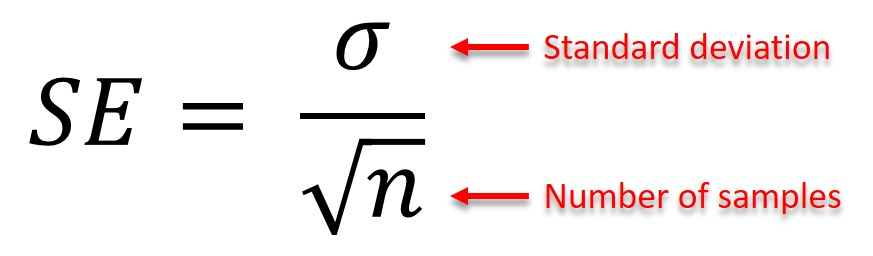# Standard Error: What It Is, Why It Matters, and How to Calculate

When you take samples from a population and calculate the means of the samples, these means will be arranged into a distribution around the true population mean.

The standard deviation of this distribution of sampling means is known as the standard error.

## Large vs. Small Standard Error

Standard error estimates how accurately the mean of any given sample represents the true mean of the population.

A larger standard error indicates that the means are more spread out, and thus it is more likely that your sample mean is an inaccurate representation of the true population mean.

On the other hand, a smaller standard error indicates that the means are closer together. Thus it is more likely that your sample mean is an accurate representation of the true population mean.

The standard error increases when the standard deviation increases. Standard error decreases when sample size increases because having more data yields less variation in your results.

## FormulaSE = standard error of the sample

σ  = sample standard deviation

n  = number of samples

## How to Calculate

Standard error is calculated by dividing the standard deviation of the sample by the square root of the sample size.

1. Calculate the mean of the total population.
2. Calculate each measurement’s deviation from the mean.
3. Square each deviation from the mean.
4. Add the squared deviations from Step 3.
5. Divide the sum of the squared deviations by one less than the sample size (n-1).
6. Calculate the square root of the value obtained from Step 5. This result gives you the standard deviation.
7. Divide the standard deviation by the square root of the sample size (n). This result gives you the standard error.
8. Subtracting the standard error from the mean / adding the standard error to the mean will give the mean ± 1 standard error.

## Example:

The values in your sample are 52, 60, 55, and 65.

• Calculate the mean of these values by adding them together and dividing by 4. (52 + 60 + 55 + 65)/4 = 58 (Step 1).
• Next, calculate the sum of the squared deviations of each sample value from the mean (Steps 2-4).
• Using the values in this example, the squared deviations are (58 – 52)^2= 36, (58 – 60)^2= 4, (58 – 55)^2=9, and (58 – 65)^2=49. Therefore, the sum of the squared deviations is 98 (36 + 4 + 9 + 49).
• Next, divide the sum of the squared deviations by the sample size minus one and take the square root (Steps 5-6). The standard deviation in this example is the square root of [98 / (4 – 1)], which is about 5.72.
• Lastly, divide the standard deviation, 5.72, by the square root of the sample size, 4 (Step 7). The resulting value is 2.86, which gives the standard error of the values in this example.

### 1. When should Standard Error be used?

We use the standard error to indicate the uncertainty around the estimate of the mean measurement. It tells us how well our sample data represents the whole population. This is useful when we want to calculate a confidence interval.

### 2. What is the Difference between Standard Error and Standard Deviation?

Standard error and standard deviation are both measures of variability, but standard deviation is a descriptive statistic that can be calculated from sample data, while standard error is an inferential statistic that can only be estimated.

Standard deviation tells us how concentrated the data is around the mean. It describes variability within a single sample. On the other hand, the standard error tells us how the mean itself is distributed.

It estimates the variability across multiple samples of a population. The formula for standard error calculates the standard deviation divided by the square root of the sample size.

References

Altman, D. G., & Bland, J. M. (2005). Standard deviations and standard errors. Bmj, 331 (7521), 903.

Zwillinger, D. (2018). CRC standard mathematical tables and formulas. chapman and hall/CRC.Saul Mcleod, PhD

BSc (Hons) Psychology, MRes, PhD, University of Manchester

Educator, Researcher

Saul Mcleod, Ph.D., is a qualified psychology teacher with over 18 years experience of working in further and higher education.Julia Simkus

Research Assistant at Princeton University